# QtiPlot - Data Analysis and Scientific Visualisation

## Signal ProcessingThe Fourier transform decomposes a function (often a function of time) or a signal into its constituent frequencies. The fast Fourier transform (FFT) is an algorithm for computing the discrete Fourier transform.

In this example of FFT performed in QtiPlot, the original signal is displayed in the bottom layer, while the amplitude-frequency plot is displayed in the top layer. The amplitude curve has been normalized, and the frequencies have been shifted to obtain a centered x-scale.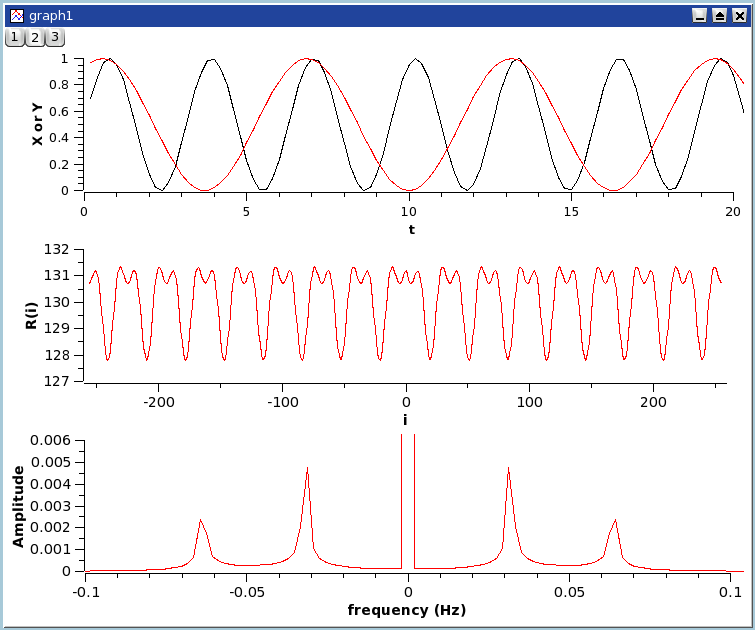The correlation function, also known as the covariance function, is used to test the similarity of two signals x(t) and y(t). The correlation of a signal with itself, called autocorrelation, is frequently used in spectral analysis.

This example shows a correlation between two sine functions. The first plot shows the two signals, the second one is the correlation function between the two signals which shows that there are correlations, and the third one is the Fourier transform which is done to extract the characteristic frequencies of the correlation function.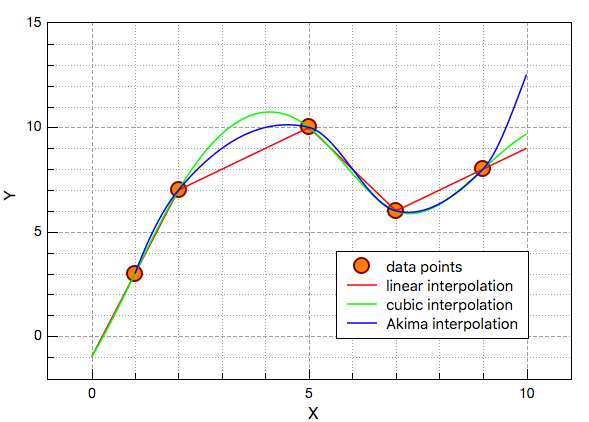Data interpolation creates a new data curve with a higher number of points generated by interpolation of your data. Three interpolation methods are available in QtiPlot.

The first is a linear method. In this case, interpolated data points are placed along the straight between every two adjacent values of your data. The second is the method of natural cubic splines. The word "natural" meaning that the second derivatives of the spline polynomials are set equal to zero at the endpoints of the interval of interpolation. The last method, Akima, is a polynomial interpolation that requires a minimum of five data points in the curve to be interpolated.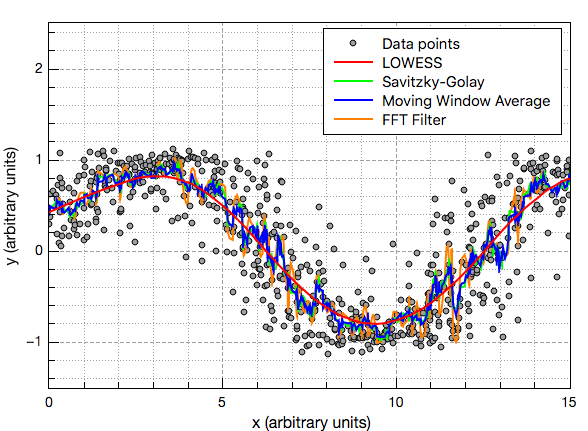There are four methods of curve smoothing available in QtiPlot: moving window average, Savitzki-Golay, LOWESS (locally weighted scatterplot smoothing) and FFT filtering. The FFT method calculates a cut-off frequency and applies a low pass filter to the data.

This example shows a comparison of these methods applied to a simulated data set, a sine curve with added white noise.

## Data fitting

QtiPlot includes quick access to the most useful functions for data fitting: linear, polynomial, exponential, logistic, Boltzmann, Gaussian, Lorentzian and Pseudo-Voigt.Linear data fitting is the process of constructing a straight line that has the best fit to a series of data points. The line equation is:

Ax + B

where A is the slope of the line and B is the Y-intercept (the point where the line crosses the Y-axis).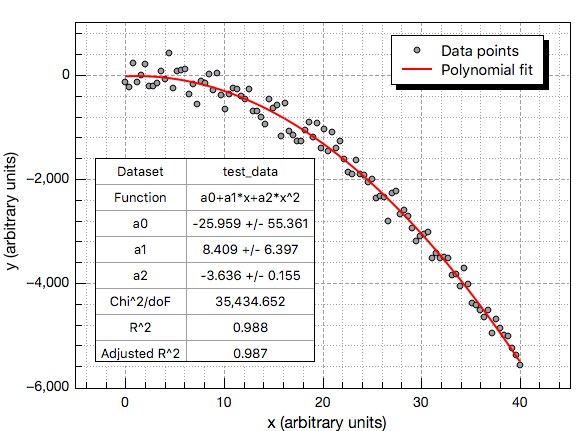Polynomial regression is a form of regression analysis in which the relationship between the independent variable x and the dependent variable y is modelled as an Nth degree polynomial.

This example shows a fit to a simulated data set with a polynom of second degree.Boltzmann and logistic models can be used to fit curves having a sigmoidal shape. The Boltzmann function used in QtiPlot is:

Y(x) = A2 + (A1 - A2)/[1 + e(x-x0)/dx]

where A1 is the low Y limit, A2 is the high Y limit, x0 is the inflexion (half amplitude) point and dx is the width.

The logistic model implemented in QtiPlot is:

Y(x) = A2 + (A1 - A2)/[1 + (x/x0)p]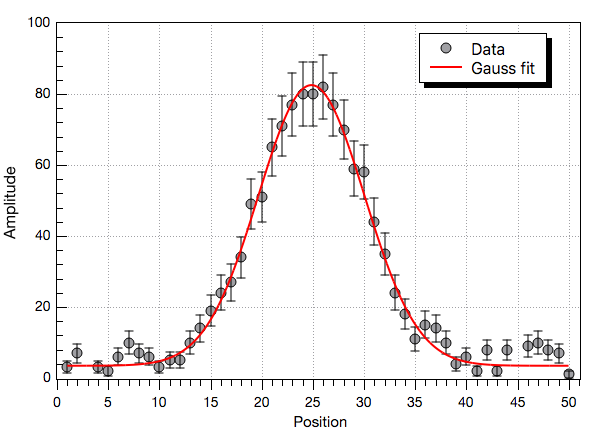The Gauss model can be used to fit a curve which has a bell shape. The function used in QtiPlot is:

Y(x) = y0 + Ae-(x - xc)2/2w2

in which A is the height, w is the width, xc is the center and y0 is the Y-values offset.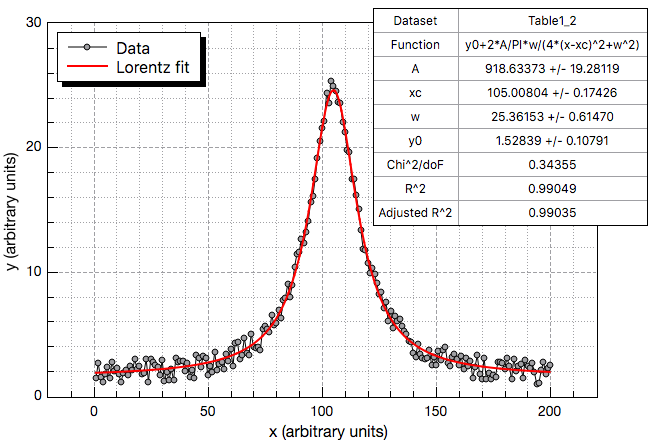In spectroscopy, the Lorentz distribution is used to describe the shape of spectral lines which are subject to homogeneous broadening in which all atoms interact in the same way with the frequency range contained in the line shape.

The function used in QtiPlot is:

Y(x) = y0 + 2Aw/[4π(x - xc)2 + w2]

in which A is the height, w is the width, xc is the center and y0 is the Y-values offset.The Pseudo-Voigt fit model is often used for calculations of experimental spectral line shapes. It is a linear combination of Gaussian and Lorentzian functions with different FWHM.

The parameters of the Pseudo-Voigt function have the following meaning: y0 is the Y-values offset, A is the area, wG is the Gaussian FWHM, wL is the Lorentzian FWHM, xc is the center and mu is a profile shape factor.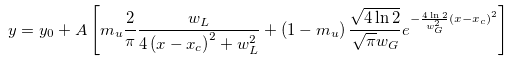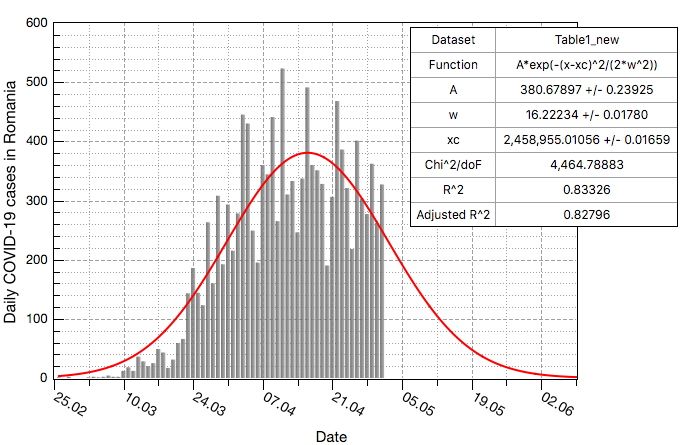Using the fit wizard tool it is possible to define your own custom fit model.

This example shows the results of a non-linear fit using a modified Gauss model with the offset term y0 set to zero:

Y(x) = Ae-(x - xc)2/2w2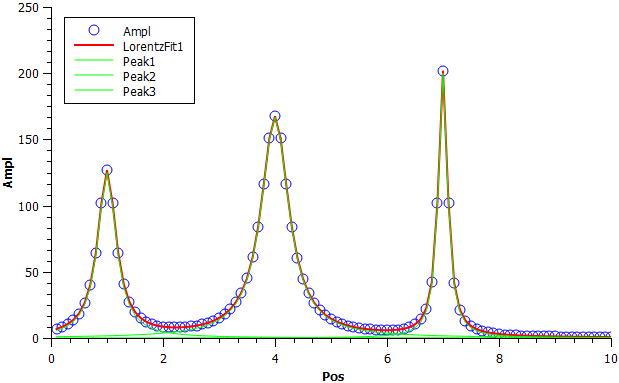The multi-peak fit tool in QtiPlot allows to fit your data points to a sum of Gaussian, Lorentzian or Pseudo-Voigt functions.

The first step is to specify the number of peaks. Then you must define the position of each peak on the curve. When the selection step ends QtiPlot pops-up a dialog that summarizes the positions of the peaks and lets you choose if you perform the fit using these parameters or if you want to open the fit wizard and refine the initial guesses before performing the fit.

## Image Analysis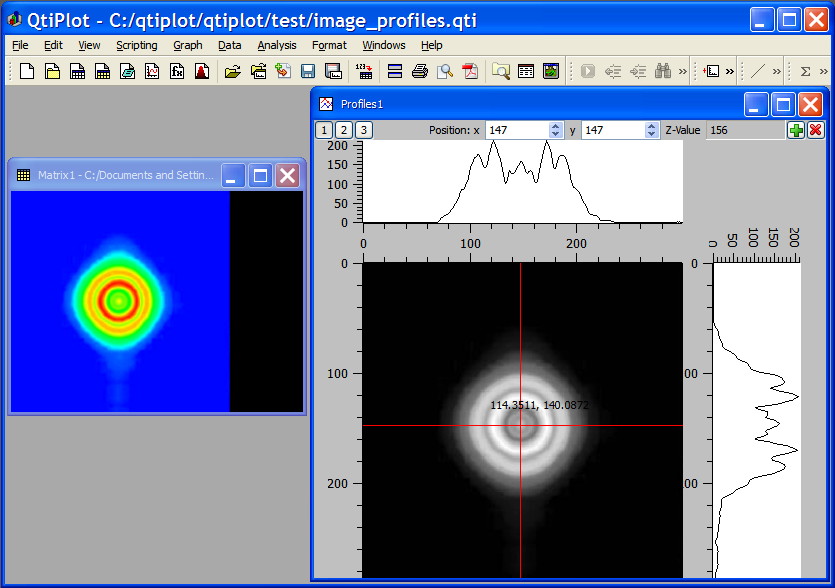The image profiles graph creates a gray scale matrix plot of the analysed image and lets you see the X and Y intensity profiles of the data pixels. The ability to control the exact position of the horizontal and vertical lines along each axis is provided.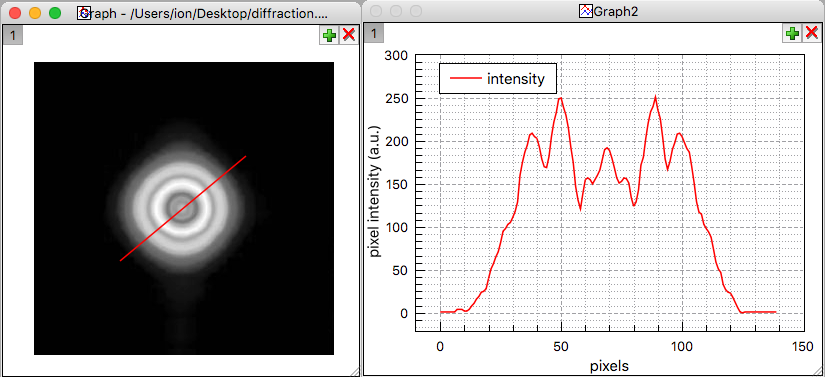The View Pixel Line Profile tool can be used to retrieve the pixel intensities from an image in a 2D plot layer. It opens an integer value dialog allowing to select the number of pixels to be averaged when calculating the pixel intensity. The selection of the pixel range from the image is done manually by drawing a line whose end points must be situated inside the image. This handy image analysis tool creates a new plot window displaying the intensity profile curve.